# Pied Flycatcher 2: Random Slopes

#### 2021-11-22

This vignette continues the example of the pied flycatchers discussed in the vignette “pied-flycatchers-1” by adding a random slope to the fixed effects model. I highly recommend that you work through the other vignette first.

# Library

First you have to load the package:

## Load package
library(dalmatian)

# Raw data

The raw data for this example is provided in the package and can be accessed with:

## Load pied flycatcher data
data(pied_flycatchers_1)

As before we will consider load as a response variable rounded to the nearest whole number and define the lower and upper bounds:

## Create variables bounding the true load
pfdata$lower=ifelse(pfdata$load==0,log(.001),log(pfdata$load-.049)) ## Warning in log(pfdata$load - 0.049): NaNs produced
pfdata$upper=log(pfdata$load+.05)

# Model

The model we consider will be the similar to the random effects model in “pied-flycatchers-1”. We will modify this model by including a random, individual slope for the effect of $$log(IVI)$$ as well as the random intercept in the mean model. The new model for the mean will be: $\mu_{ij}=\beta_0 + \beta_1 \mathrm{log(IVI)_{ij}} + \beta_2 \mathrm{broodsize}_i + \beta_3 \mathrm{sex}_i + \epsilon_{1i} + \epsilon_{2i} \mathrm{log(IVI)_{ij}}$ For convenience, we will also simplify the model of the dispersion by assuming that the variance is constant across all observations. This can be done by setting: $\log(\phi_{ij})=\psi_0$.

The objects defining the new mean and dispersion components are specified as:

# Random component of mean
mymean=list(fixed=list(name="alpha",
formula=~ log(IVI) + broodsize + sex,
priors=list(c("dnorm",0,.001))),
random=list(name="epsilon",
formula=~-1 + indidx + indidx:log(IVI)))

# Random component of dispersion
mydisp=list(fixed=list(name="psi",
formula=~1,
priors=list(c("dnorm",0,.001))),
link="log")

## Running the Model with dalmatian

The new model can now be run using dalmatian in exactly the same way as above. However, we will make one change. In order to shorten the convergence time, we will base initial values on the results of the fixed effects model and then provide these as input. In particular, we will define initial values for the fixed effects of both the mean and dispersion components by taking the values from the final iterations of each of the chains run for the fixed effects model. For convenience, we set the random effects variances equal to 1 in all three chains. Note that the chains have been run for a relatively small number of iterations and heavily subsampled to satisfy the size requirements for packages on . Neither of these is recommended in practice.

## Set working directory
## By default uses a system temp directory. You probably want to change this.
workingDir <- tempdir()

## Define list of arguments for jags.model()

## Define list of arguments for coda.samples()
cs.args <- list(n.iter=1000)

## Run the model using dalmatian
pfmcmc3 <- dalmatian(df=pfdata,
mean.model=mymean,
dispersion.model=mydisp,
jags.model.args=jm.args,
coda.samples.args=cs.args,
rounding=TRUE,
lower="lower",
upper="upper",
debug=FALSE)

## Results

Once again, we can use the wrapper functions provided with dalmatian to conduct convergence diagnostics and compute summary statistics for the posterior distribution from which we can make inference. Note that the chains have been thinned prior to creating the traceplots in order to reduce the size of the package.

1. Convergence diagnostics
## Compute convergence diagnostics
pfconvergence3 <- convergence(pfmcmc3,raftery=list(r=.01))
## Gelman-Rubin diagnostics
pfconvergence3$gelman ## Potential scale reduction factors: ## ## Point est. Upper C.I. ## alpha.(Intercept) 1.00 1.01 ## alpha.log(IVI) 1.00 1.01 ## alpha.broodsizeIncreased 1.01 1.02 ## alpha.sexMale 1.02 1.06 ## psi.(Intercept) 1.00 1.01 ## sd.epsilon.indidx 1.01 1.04 ## sd.epsilon.indidx:log(IVI) 1.01 1.03 ## Raftery diagnostics pfconvergence3$raftery
##
## Quantile (q) = 0.025
## Accuracy (r) = +/- 0.005
## Probability (s) = 0.95
##
## You need a sample size of at least 3746 with these values of q, r and s
## Effective sample size
pfconvergence3$effectiveSize ## alpha.(Intercept) alpha.log(IVI) ## 359.1056 1548.6355 ## alpha.broodsizeIncreased alpha.sexMale ## 172.0363 143.7425 ## psi.(Intercept) sd.epsilon.indidx ## 538.2383 447.4075 ## sd.epsilon.indidx:log(IVI) ## 122.9022 1. Traceplots ## Generate traceplots pftraceplots3 <- traceplots(pfmcmc3, nthin = 20, show = FALSE) ## Fixed effects for mean pftraceplots3$meanFixed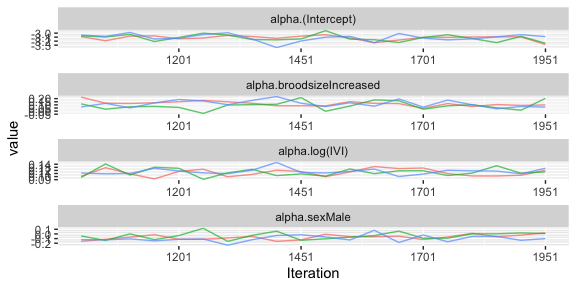## Fixed effects for dispersion
pftraceplots3$dispersionFixed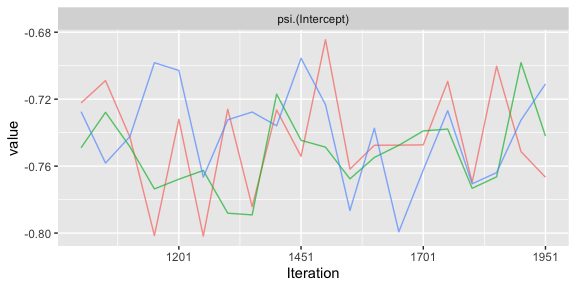## Random effects variances for mean pftraceplots3$meanRandom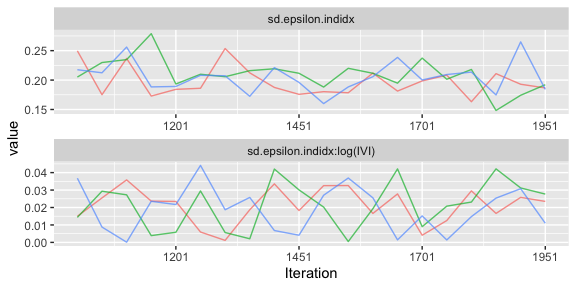## Random effects variances for dispersion
pftraceplots3$dispersionRandom ## NULL 1. Numerical summaries ## Compute numerical summaries pfsummary3 <- summary(pfmcmc3) ## Print numerical summaries pfsummary3 ## ## Iterations = 1001:2000 ## Thinning interval = 1 ## Number of chains = 3 ## Sample size per chain = 1000 ## ## Posterior Summary Statistics for Each Model Component ## ## Mean Model: Fixed Effects ## Mean Median SD Lower 95% Lower 50% Upper 50% Upper 95% ## (Intercept) -3.11 -3.10 0.09 -3.28 -3.17 -3.05 -2.93 ## log(IVI) 0.11 0.12 0.01 0.09 0.11 0.13 0.14 ## broodsizeIncreased 0.10 0.10 0.07 -0.02 0.04 0.13 0.24 ## sexMale -0.08 -0.08 0.07 -0.21 -0.13 -0.04 0.05 ## ## Mean Model: Random Effects ## Mean Median SD Lower 95% Lower 50% Upper 50% Upper 95% ## indidx 0.21 0.21 0.03 0.14 0.18 0.23 0.27 ## indidx:log(IVI) 0.02 0.02 0.01 0.00 0.01 0.03 0.04 ## ## Dispersion Model: Fixed Effects ## Mean Median SD Lower 95% Lower 50% Upper 50% Upper 95% ## (Intercept) -0.75 -0.75 0.03 -0.8 -0.77 -0.73 -0.69 1. Graphical summaries ## Generate caterpillar pfcaterpillar3 <- caterpillar(pfmcmc3,show = FALSE) ## Fixed effects for mean pfcaterpillar3$meanFixed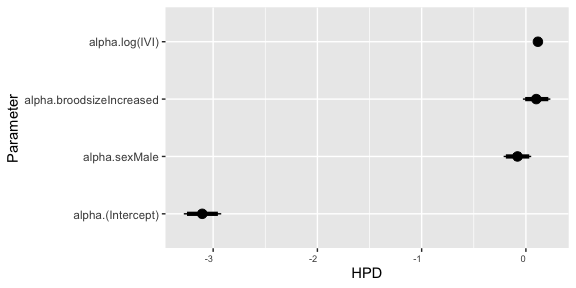## Fixed effects for dispersion
pfcaterpillar3$dispersionFixed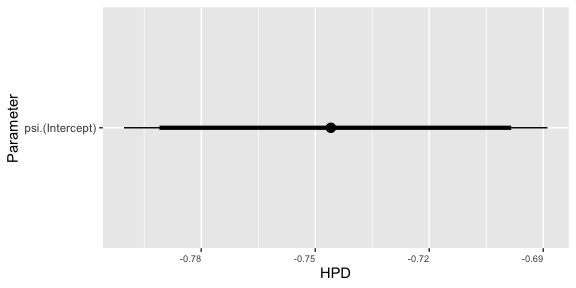The convergence diagnostics and traceplots suggest that the chains should be run for longer. In particular, the effective sample size for the variance of the random slopes is very small, but we will proceed with inference for illustration. In the numerical summaries we see that the estimated variance of the random slopes is very small, 0.0204748 with 95% credible interval (2.7611746^{-5},0.040385). This suggests that there is very little inter-individual variation in the effect of log(IVI). Estimates of the individual random effects (predictions for frequentists) can be obtained from the function ranef(). This function computes posterior summary statistics for the individual random effects. In this case, the random effects for the mean include both the intercept and the effect of log(IVI). The following code computes the summary statistics and plots the predicted values of the random slopes with HPD 95% confidence intervals. ## Compute summary statistics for random effects pfranef3 <- ranef(pfresults3) ## Load ggplot2 library(ggplot2) ## Identify number of individuals nind <- nlevels(pfdata$indidx)

## Plot predicted random slopes
ggplot(data=as.data.frame(pfranef3\$mean[nind+(1:nind),]),aes(x=1:nind,y=Mean)) +
geom_point() +
geom_errorbar(aes(ymin=Lower 95%,ymax=Upper 95%)) +
geom_abline(intercept=0,slope=0)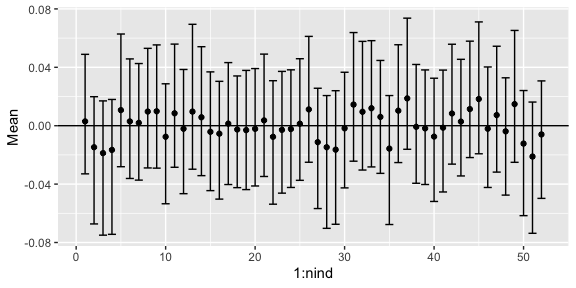Note that the 95% credible intervals for all of the values include 0. This is further evidence that the effect of log(IVI) does not vary significantly between the individuals.